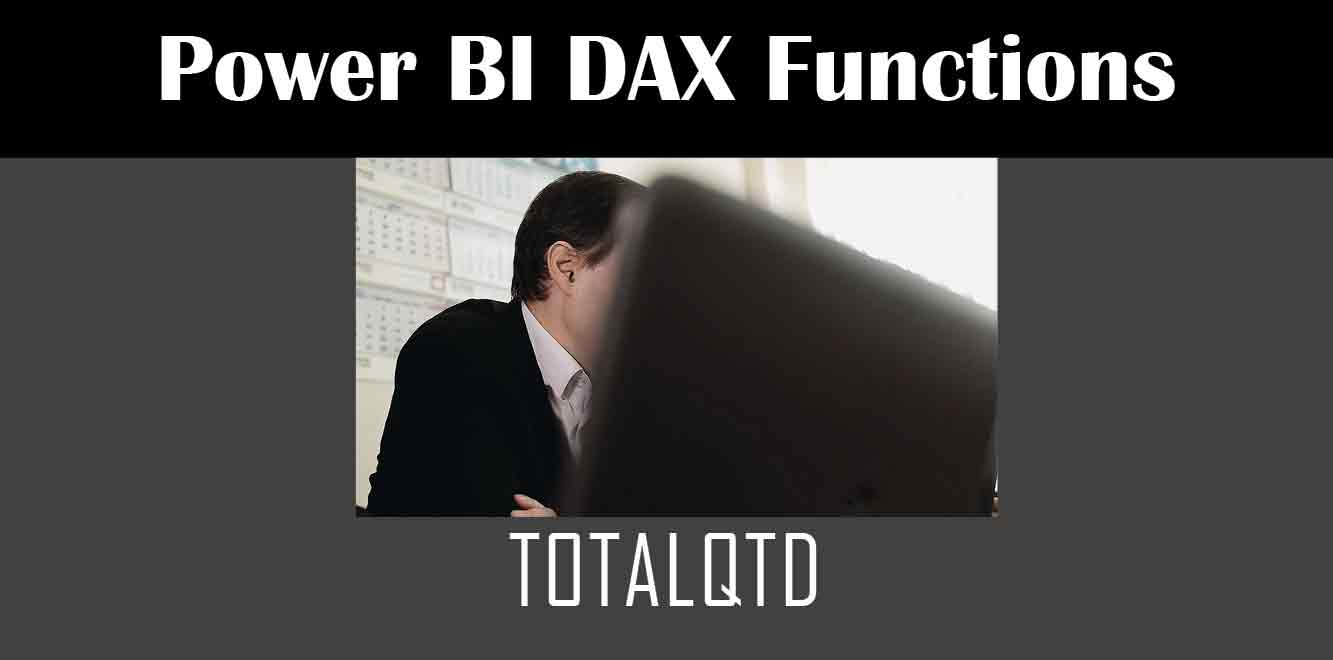# TOTALQTD

## Y## Syntax of TOTALQTD Function

The TOTALQTD function returns the total of a measure for all dates from the beginning of the quarter until the current date. The syntax of the TOTALQTD function is as follows:

TOTALQTD(❰expression❱, ❰date_column❱)

``` The first parameter is the expression or measure to be calculated, and the second parameter is the date column that contains the dates for which the calculation will be performed. Example of TOTALQTD Function Suppose you have a sales table with the following columns: date, region, product, and sales. To calculate the total sales from the beginning of the quarter until the current date, you can use the following DAX formula: ```

TOTALQTD(SUM(sales), date)

``` The above formula will sum up all the sales for the dates from the beginning of the quarter until the current date. Using TOTALQTD with Filters You can also use the TOTALQTD function with filters. For example, if you want to calculate the total sales for a specific region from the beginning of the quarter until the current date, you can use the following DAX formula: ```

TOTALQTD(CALCULATE(SUM(sales), region = “North”), date)

``` The above formula will calculate the total sales for the North region from the beginning of the quarter until the current date. Using TOTALQTD with Multiple Measures You can also use the TOTALQTD function with multiple measures. For example, if you want to calculate the total sales and the total profit from the beginning of the quarter until the current date, you can use the following DAX formula: ```

TOTALQTD(SUM(sales), date) + TOTALQTD(SUM(profit), date)

``` The above formula will calculate the total sales and the total profit from the beginning of the quarter until the current date and add them up. The TOTALQTD function in Power BI DAX is a powerful tool for calculating the total of a measure from the beginning of the quarter until the current date. By properly using filters and multiple measures, you can create more complex calculations. We hope this article has provided you with a good understanding of how to use the TOTALQTD function in Power BI. Power BI DAX Training Courses by G Com Solutions (0800 998 9248)Power BI DAX Intensive Training Course £1,050.00 – £26,550.00 Select optionsContinue Loading DonePower BI DAX Introduction £395.00 – £9,750.00 Select optionsContinue Loading DonePower BI DAX Intermediate £395.00 – £9,750.00 Select optionsContinue Loading DonePower BI DAX Advanced £395.00 – £9,750.00 Select optionsContinue Loading Done Upcoming Courses Contact Us Subject Your Name (required) Company/Organisation Email (required) Telephone Training Course(s) Power BI Intensive TrainingPower BI introduction Power BI IntermediatePower BI AdvancedDAXPower Query MPower BI CertificationPower BI AdministrationPower PlatformPower AutomatePower AppsOTHER Your Message Upload Example Document(s) (Zip multiple files) ```
``` ```
``` ```
``` ```
``` ```
``` ```